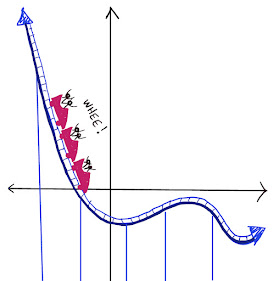## Thursday, April 11, 2019

### Domino Square Puzzle

There are 28 different dominoes in a set.  In the puzzle below, four dominoes from a set are placed in the shape of a square.  This is a "domino square."

We can make a puzzle out of a domino square by trying to arrange it so that we have the same number of "pips" (or dots) along each edge of the square.  It could be any number, it just has to be the same one each side.

Notice that the domino square below almost works -- it has 10 pips along three of the four edges, but the botton edge has only 7 pips.

Can you fix this square by replacing one of the dominoes so that every side has 10 pips?  Remember that the dominoes must all be different.

How many domino squares can you make with a single set of dominoes?

For the solution, click "Read More" below.

Solution

This is the solution to the puzzle above.  It is also possible to use a full set of 28 dominoes to make exactly 7 domino squares.